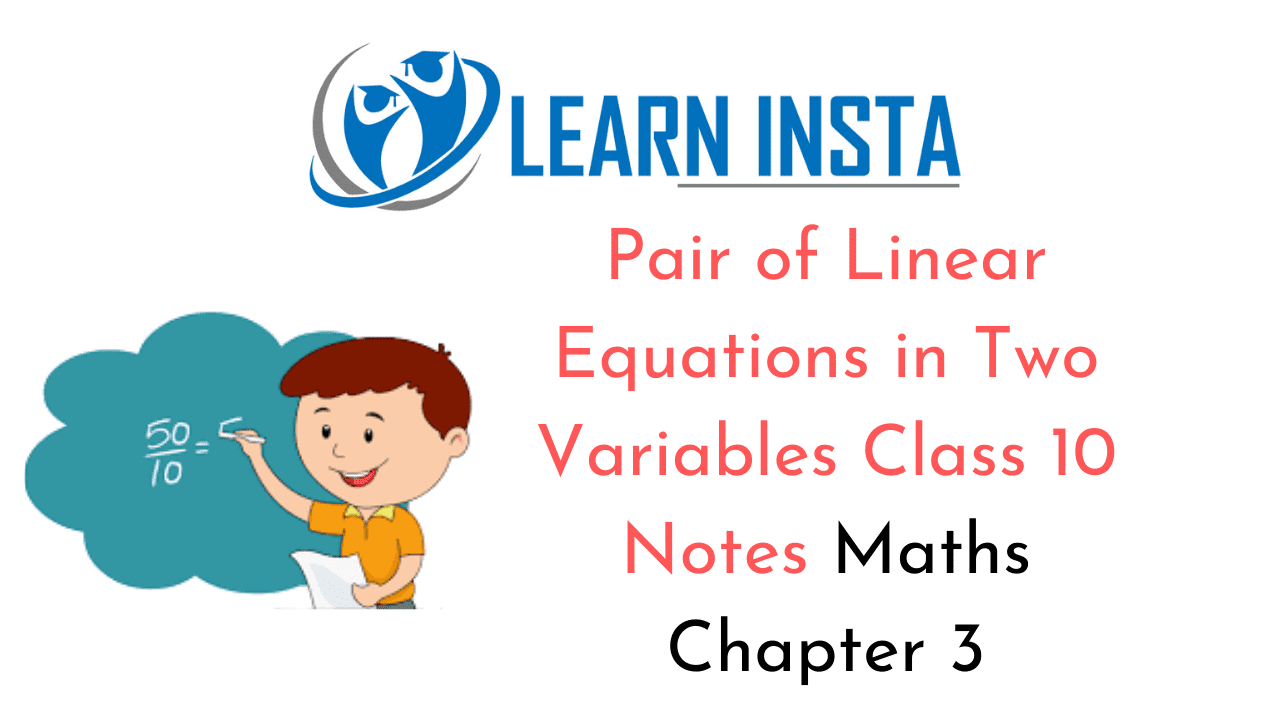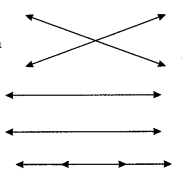On this page, you will find Pair of Linear Equations in Two Variables Class 10 Notes Maths Chapter 3 Pdf free download. CBSE NCERT Class 10 Maths Notes Chapter 3 Pair of Linear Equations in Two Variables will seemingly help them to revise the important concepts in less time.

## CBSE Class 10 Maths Chapter 3 Notes Pair of Linear Equations in Two Variables

### Pair of Linear Equations in Two Variables Class 10 Notes Understanding the Lesson

Two linear equations in the same two variables are called a pair of linear equations in two variables. The most general form of a pair of linear equation is
a1x + b1y + c1 = 0
a2x+ b2y + c2 = 0
where a1,a2, b1,b2, c1 c2 are real numbers. For the pair of linear equations, the following situations can arise:
(i) $$\frac{a_{1}}{a_{2}} \neq \frac{b_{1}}{b_{2}}$$ In this case, the pair of linear equations is consistent.

(ii) $$\frac{a_{1}}{a_{2}}=\frac{b_{1}}{b_{2}} \neq \frac{c_{1}}{c_{2}}$$ The pair of linear equations in inconsistent.

(iii) $$\frac{a_{1}}{a_{2}}=\frac{b_{1}}{b_{2}}=\frac{c_{1}}{c_{2}}$$ The pair of linear equations is consistent.

2. A pair of linear equations in two variables, can be represented, and solved by the

• Graphical method
• Algebraic method

3. Graphical Method: The graph of a pair of linear equations in two variables is represented by two lines, following three possibilities can occur.

• Two lines intersect at one point, then that point gives the unique solution of the two equations and the pair of equations is consistent.
• Two lines will not intersect, i.e. they are parallel, the pair of linear equations is inconsistent and the pair of equations will have no solution.• The graph will be a pair of coincident lines. Each point on the lines will be a solution, so the pair of equations will have infinitely many solution and is consistent.

4. Algebraic Method: A pair of linear equations can be solved by any of the following three methods:

• Substitution method
• Elimination method
• Cross-multiplication method

5. Graphical Method of Solution of a pair of Linear Equations:

If the lines represented by the pair of linear equations in two variables are given by
a1x + b1y + c1 = 0
a2x+ b2y + c2 = 0

Following are the cases:

(i) If $$\frac{a_{1}}{a_{2}} \neq \frac{b_{1}}{b_{2}}$$ then the lines are intersecting lines and intersect at one point. In this case, the pair of  linear equations in consistent.

(ii) If $$\frac{a_{1}}{a_{2}}=\frac{b_{1}}{b_{2}}=\frac{c_{1}}{c_{2}}$$, then the lines are coincident. In this case, the pair of linear equation is consistent  (dependent)

(iii) If $$\frac{a_{1}}{a_{2}}=\frac{b_{1}}{b_{2}} \neq \frac{c_{1}}{c_{2}}$$ then the lines are parallel to each other. In this case, the pair of linear equations inconsistent.